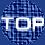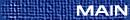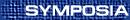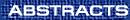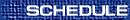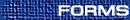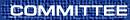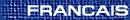Plenary Speakers / Conférenciers principaux GEORGIA BENKART, Department of Mathematics, University of Wisconsin-Madison, Madison, Wisconsin  53706, USA Canadian Lie algebras Finite-dimensional complex simple Lie algebras and affine Lie algebras have played a prominent role in mathematics and physics. Kac-Moody Lie algebras, Lie algebras graded by finite root systems, and extended affine Lie algebras all are natural generalizations of them. Our understanding of these Lie algebras has been greatly enriched by the work of Canadian mathematicians. This talk will survey results on these general classes of Lie algebras. ZOE CHATZIDAKIS, CNRS, Université Paris 7, France Model theory of generic difference fields A difference field is a field with a distinguished automorphism. A generic difference field is a difference field such that every system of difference equations which has a solution in a difference field extension, has a solution in the field. In the first part of the talk I will state the main model-theoretic results obtained on these fields and explain their significance and importance. In the second part of the talk, I will mention some applications obtained by Hrushovski to the solution of diophantine problems (e.g., the Manin-Mumford conjecture and the Jacobi conjecture for difference fields). I will also mention some intriguing questions, which lie at the boundary of model theory and diophantine geometry. GEOFFREY GRIMMETT, University of Cambridge, Statistical Laboratory, CMS, Wilberforce Road, Cambridge  CB3 0WB, UK Conformality and universality What is the Hausdorff dimension of the perimeter of Brownian motion? What is the exponent in the formula for the number of self-avoiding walks? Prove the physicists' conjectures for exact values of critical exponents for percolation. Derive a rigorous theory of universality for lattice systems. (All in two dimensions.) Recent insights threaten to answer such challenges, and more. (The answer to the first is definitely 4/3.) Schramm has shown how Loewner's work on conformal maps provides a family of canonical random processes which `should' be the limits of such spatial systems in the limit of small mesh size. Smirnov has justified the `should' for site percolation on the triangular lattice. We shall outline these famous problems, and the (proposed) programme of solution. This a beautiful story of the interaction of mathematical disciplines, and involves probability, mathematical physics, conformal functions, and combinatorics. BARRY SIMON, Department of Mathematics, Caltech, Pasadena, California  91125, USA Singular continuous spectra for Schrodinger operators I'll begin by reviewing the decomposition of measures on the real line into spectral types and then overview the recent literature on the occurrence of ``exotic'' spectral types for one dimensional operators of the form Hu = -u¢¢+qu.